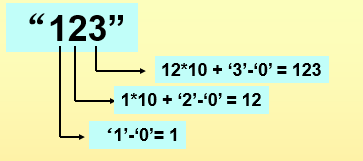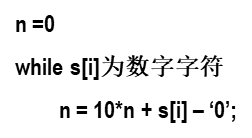• 将一个字符串转换成一个整数，要求不能使用字符串转换整数的库函数。 数值为0或者字符串不是一个合法的数值则返回0
• 将数字字符串转换成整数
问题
将数字字符串转换成整数
算法分析算法步骤如下：算法实现
#include <stdio.h>
int atoi(char s[ ]);
main()
{
char s;
scanf("%s", s);
printf("%d\n", atoi(s));
}
int atoi(char s[ ])
{
int i, n, sign;
for(i=0; s[i] = = ' ' || s[i] = = '\n' || s[i] = = '\t'; i++)
; 	/* skip white space */
sign = 1;
if(s[i] = = '+' || s[i] = = '-')
sign = (s[i++] = = '+')?1:-1;
for(n=0; s[i] >= '0' && s[i] <= '9'; i++)
n = 10*n + s[i] – '0';
return ( sign * n);
}


展开全文C语言
• 请你来实现一个 atoi 函数，使其能将字符串转换成整数。 首先，该函数会根据需要丢弃无用的开头空格字符，直到寻找到第一个非空格的字符为止。接下来的转化规则如下： 如果第一个非空字符为正或者负号时，则将该符号...
请你来实现一个 atoi 函数，使其能将字符串转换成整数。
首先，该函数会根据需要丢弃无用的开头空格字符，直到寻找到第一个非空格的字符为止。接下来的转化规则如下：
如果第一个非空字符为正或者负号时，则将该符号与之后面尽可能多的连续数字字符组合起来，形成一个有符号整数。 假如第一个非空字符是数字，则直接将其与之后连续的数字字符组合起来，形成一个整数。 该字符串在有效的整数部分之后也可能会存在多余的字符，那么这些字符可以被忽略，它们对函数不应该造成影响。 注意：假如该字符串中的第一个非空格字符不是一个有效整数字符、字符串为空或字符串仅包含空白字符时，则你的函数不需要进行转换，即无法进行有效转换。
在任何情况下，若函数不能进行有效的转换时，请返回 0 。
提示：
本题中的空白字符只包括空格字符 ’ ’ 。 假设我们的环境只能存储 32 位大小的有符号整数，那么其数值范围为 [−231, 231 − 1]。如果数值超过这个范围，请返回 INT_MAX (231 − 1) 或 INT_MIN (−231) 。
示例 1:
输入: “42” 输出: 42 示例 2:
输入: " -42" 输出: -42 解释: 第一个非空白字符为 ‘-’, 它是一个负号。 我们尽可能将负号与后面所有连续出现的数字组合起来，最后得到 -42 。 示例 3:
输入: “4193 with words” 输出: 4193 解释: 转换截止于数字 ‘3’ ，因为它的下一个字符不为数字。 示例 4:
输入: “words and 987” 输出: 0 解释: 第一个非空字符是 ‘w’, 但它不是数字或正、负号。 因此无法执行有效的转换。 示例 5:
输入: “-91283472332” 输出: -2147483648 解释: 数字 “-91283472332” 超过 32 位有符号整数范围。 因此返回 INT_MIN (−2^31) 。
C++ 初稿
class Solution {
public:
int myAtoi(string s) {
int i=0;
bool fu = false;
bool chara = false;
vector <int> result;
if(!s)
return 0;

while(s[i]==' ')
{
i++;
}
if(s[i]=='-'&&fu==false&&chara==false)
{
fu=true;
chara = true;
i++;
}
if(s[i]=='+'&&chara==false)
{
chara = true;
i++;
}
while(s[i]=='0')
{
i++;
chara=true;
}
while(i<s.size())
{

if(s[i]>='0'&&s[i]<='9')
{
chara = true;
result.push_back(s[i]-'0');
}
else
break;
i++;

}

long sum=0;
int len = result.size();
i=0;
if(len>10&&fu==false)
return INT_MAX;
if(len>10&&fu==true)
return INT_MIN;
while(i<result.size())
{
sum += pow(10,len-1)*(result[i]);
i++;
len--;
}
if(fu)
sum = -sum;
if(sum<INT_MIN)
return INT_MIN;
if(sum>INT_MAX)
return INT_MAX;

return sum;
}
};

优化后
class Solution {
public:
int myAtoi(string s) {
int i=0;
bool fu = false;
while(s[i]==' ')
{
i++;
}
if(s[i]=='-')
{
fu=true;
}
if(s[i]=='+'||s[i]=='-')
{
i++;
}
int sum=0;
while(i<s.size()&&isdigit(s[i]))
{

int r = s[i]-'0';

if(sum>INT_MAX/10||(INT_MAX/10==sum&&r>7))//也可用double，不过会慢。
return fu? INT_MIN:INT_MAX;
sum = sum*10 + r;
i++;
}

return fu? -sum:sum;
}
};

答案用的自动机DFAclass Automaton {
string state = "start";
unordered_map<string, vector<string>> table = {
{"start", {"start", "signed", "in_number", "end"}},
{"signed", {"end", "end", "in_number", "end"}},
{"in_number", {"end", "end", "in_number", "end"}},
{"end", {"end", "end", "end", "end"}}
};

int get_col(char c) {
if (isspace(c)) return 0;
if (c == '+' or c == '-') return 1;
if (isdigit(c)) return 2;
return 3;
}
public:
int sign = 1;
long long ans = 0;

void get(char c) {
state = table[state][get_col(c)];
if (state == "in_number") {
ans = ans * 10 + c - '0';
ans = sign == 1 ? min(ans, (long long)INT_MAX) : min(ans, -(long long)INT_MIN);
}
else if (state == "signed")
sign = c == '+' ? 1 : -1;
}
};

class Solution {
public:
int myAtoi(string str) {
Automaton automaton;
for (char c : str)
automaton.get(c);
return automaton.sign * automaton.ans;
}
};


附一个一行的python代码
class Solution:
def myAtoi(self, s: str) -> int:
return max(min(int(*re.findall('^[\+\-]?\d+', s.lstrip())), 2**31 - 1), -2**31)


由这个python代码想到了正则表达式 C++的正则表达式方法
class Solution {
public:
int sign = 1;
int myAtoi(string s) {
s.erase(0,s.find_first_not_of(" "));
regex e("^[\\+\\-]?\\d+");
smatch m;
bool found = regex_search(s, m, e);
int ans = 0;
string resu = m.str(0);
if(resu=='-')
{
sign = -1;
}
if(resu=='+'||resu=='-')
{
resu.erase(0,1);
}
for(char c : resu)
{
int num = c - '0';
if(ans>INT_MAX/10||(ans==INT_MAX/10&&num>7))
{
return sign==1? INT_MAX:INT_MIN;
}
ans = ans * 10 + num;
}
return sign * ans;
}
};

展开全文c++ 算法
• ## Java实现字符串转换成整数

万次阅读 多人点赞 2019-07-21 14:52:11
输入一个由数字组成的字符串，请把它转换成整数并输出。例如，输入字符串“123”，输出整数123。 请写出一个函数实现该功能，不能使用库函数。 2 解决方案 解答本问题的基本思路：从左至右扫描字符串中的每个字符，...
1 问题描述 输入一个由数字组成的字符串，请把它转换成整数并输出。例如，输入字符串“123”，输出整数123。
请写出一个函数实现该功能，不能使用库函数。
2 解决方案 解答本问题的基本思路：从左至右扫描字符串中的每个字符，把之前扫描得到的数字乘以10，再加上当前字符表示的数字。
但是，基本思路是这样，还要注意以下几点：
（1）最好判断一下输入是否为空。
（2）如果字符串的第一个字符是‘-’号，最终得到的整数必为负整数。
（3）输入的字符串中不能含有不是数字的字符。
（4）输入的字符串不能太长，否则转换成整数后会导致整数溢出。
package com.liuzhen.string_1;

import java.util.Scanner;

public class StringToInt {
public static int Max_INT = Integer.MAX_VALUE;
public static int Min_INT = Integer.MIN_VALUE;

public int getStringToInt(String A){
char[] arrayA = A.toCharArray();
int n = 0;
if(A.equals("") || A.equals(null))     //判断输入是否为空
return 0;
int i = 0;
while(arrayA[i] == ' ')   //处理字符串首位的空格
i++;
int sign = 1;   //用于判定输入字符串数字的正负，初始化为1表示为正数
if(arrayA[i] == '+' || arrayA[i] == '-'){
if(arrayA[i] == '-')
sign = -1;
i++;
}
while(i < arrayA.length && Character.isDigit(arrayA[i])){  //确定是数字0~9才执行循环
int c = arrayA[i] - '0';
//当输入字符串表示数为正数，且大于Max_INT
if(sign > 0 && (n > Max_INT/10 || (n == Max_INT/10 && c > Max_INT%10))){
n = Max_INT;
break;
}
//当输入字符串表示数为负数，且小于Min_INT
if(sign < 0 && (n + Min_INT/10 > 0 || (n + Min_INT/10 == 0 && c + Min_INT%10 > 0))){
n = Min_INT;
break;
}
//把之前得到的数字乘以10，再加上 当前字符表示的数字
n = n*10 + c;
i++;
}

return sign > 0 ? n : -n;
}
public static void main(String[] args){
StringToInt test = new StringToInt();
Scanner in = new Scanner(System.in);
System.out.println("请输入一个由数字组成的字符串：");
String A = in.nextLine();
int result = test.getStringToInt(A);
System.out.println("整数result = "+result);
}
}

运行结果：
请输入一个由数字组成的字符串：
-1000
整数result = -1000

请输入一个由数字组成的字符串：
+100000
整数result = 100000

请输入一个由数字组成的字符串：
a1212
整数result = 0

请输入一个由数字组成的字符串：
整数result = 2147483647

请输入一个由数字组成的字符串：
-10000000000
整数result = -2147483648

展开全文Java 字符串 转换
• 请你来实现一个 atoi 函数，使其能将字符串转换成整数。 首先，该函数会根据需要丢弃无用的开头空格字符，直到寻找到第一个非空格的字符为止。 当我们寻找到的第一个非空字符为正或者负号时，则将该符号与之后面...

剑指offer第一章的例子，据说是微软的面试题，发现自己又躺枪了。字符串处理有多烦人不用我多说了吧。

//基础版代码
int StrToInt(char* string)
{
int number = 0;
while (*string != 0){
number = number * 10 + *string - '0';
++string;
}
return number;
}

需要考虑的特殊情况有： 1.输入字符串有非数字字符和正负号； 2.考虑到最大整数和最小的负整数以及溢出； 3.考虑到当输入字符串不能转换为整数时该如何进行错误提示； 4.有空白字符应该跳过；
这与LeetCode上的一道题是一样的：

请你来实现一个 atoi 函数，使其能将字符串转换成整数。 首先，该函数会根据需要丢弃无用的开头空格字符，直到寻找到第一个非空格的字符为止。  当我们寻找到的第一个非空字符为正或者负号时，则将该符号与之后面尽可能多的连续数字组合起来，作为该整数的正负号；假如第一个非空字符是数字，则直接将其与之后连续的数字字符组合起来，形成整数。  该字符串除了有效的整数部分之后也可能会存在多余的字符，这些字符可以被忽略，它们对于函数不应该造成影响。  注意：假如该字符串中的第一个非空格字符不是一个有效整数字符、字符串为空或字符串仅包含空白字符时，则你的函数不需要进行转换。  在任何情况下，若函数不能进行有效的转换时，请返回 0。  说明： 假设我们的环境只能存储 32 位大小的有符号整数，那么其数值范围为 [−231, 231 − 1]。如果数值超过这个范围，请返回 INT_MAX (231 − 1) 或 INT_MIN (−231) 。

代码如下：
int myAtoi(string str){
bool negative = false; //适用于没出现正负号默认为正数的情况；
int index = 0; //索引；
long long result = 0;

while (index < str.size() && str[index] == ' '){
index++;
} //指向第一个非空格字符；

if (index == str.size()){ //字符串全部为空字符
return 0;
}

if (str[index] == '-'){ //正负号判定;
negative = true;
index++;
}
else if (str[index] == '+'){
negative = false;
index++;
}

while (index < str.size() && isdigit(str[index])){ //没有到字符串尾且仍然是数字；
result = result * 10 + str[index++] - '0';
if (result >= 2147483648){ //int溢出判定；
if (negative) return INT_MIN;
else return INT_MAX;
}
}
return negative ? result * (-1) : result; //根据flag negative决定返回的是整数还是负数；
}

这不禁让我想起了LeetCode里的一道类似的题

请实现一个函数用来判断字符串是否表示数值（包括整数和小数）。例如，字符串"+100"、“5e2”、"-123"、“3.1416”、“0123"及”-1E-16"都表示数值，但"12e"、“1a3.14”、“1.2.3”、"±5"及"12e+5.4"都不是。

唯一的区别是这道题里包括了小数和科学计数法，并且只需要判断字符串表示的是不是数值，而不需要真正的返回一个数值，所以可以省去溢出判定的步骤。
这类问题大概是三个思路：
根据编译原理中DFA的知识，画出状态转移表，再使用表驱动的方法，来判断是否可以从字符串中接受到合法的数值（整数和小数）。通常来说，构造DFA的一般流程是：先写出句型的正规表达式，再根据正规表达式画出NDFA，最后进行NDFA的确定化，画出状态转移表。但是这个过程比较繁琐，我花了大半晚上重温了一下编译原理心态爆找。不过这个自动机也比较简单，所以看LeetCode上的题解大家往往表示要啥自行车直接手撸DFA，然后再画出状态转移表。此处可以参考这个题解：表驱动法。字符串匹配的问题，很难不想到正则表达式。 根据题意写出符合格式的正则表达式为：’^[\+\-]?(\d+.\d+|.\d+|\d+.|\d+)(e[\+\-]?\d+)?\$’； C++可以使用regex库，Python可以使用re库，在原字符串中进行模式匹配即可。最常见的思路就是根据特征来进行判定，一般是从头到尾扫描，判断是否符合条件。如果成功扫描结束则返回True，如果中途有违反条件的地方则返回True； 代码：
class Solution {
public:
bool isNumber(string &s)
{
int i = 0;

//skip the whilespace;
for (; s[i] == ' '; i++);

//check the sign if exist;
if (s[i] == '+' || s[i] == '-') i++;

//a trick
int n_nm, n_pt;
for (n_nm = 0, n_pt = 0; isdigit(s[i]) || s[i] == '.'; i++){
s[i] == '.' ? n_pt++ : n_nm++;
}
if (n_pt > 1 || n_nm < 1) return false;

//check the exponent if exist
if (s[i] == 'e'){
i++;
if (s[i] == '+' || s[i] == '-') i++;

int n_nm = 0;
for (; isdigit(s[i]); i++, n_nm++);
if (n_nm < 1) return false;
}

//skip the trailing whitespaces
for (; s[i] == ' '; i++);

return s[i] == 0;
}
};

展开全文leetcode c++
• 合数字要求时返回0)，要求不能使用字符串转换整数的库函数。 数值为0或者字符串不是 一个合法的数值则返回0。 输入描述: 输入一个字符串,包括数字字母符号,可以为空 输出描述: 如果是合法的数值表达则返回该数字，...剑指offer C++
• 将一个字符串转换成一个整数(实现Integer.valueOf(string)的功能，但是string不符合数字要求时返回0)，要求不能使用字符串转换整数的库函数。 数值为0或者字符串不是一个合法的数值则返回0。 输入描述: 输入一个字符...
• Python 把字符串转换成整数 绝对通过的方法补码 正则表达式 python
• 按照题目要求将一个字符串转换成一个整数，首先忽略掉前面的“+”“-”后，主要的逻辑就是将字符串中的元素一个一个拿出来转换成整数然后循环乘10后进行累加就可以拿到转换之后的整数。 而一个字符a转换成整数只要他...C++
• 题目：字符串转换整数。注意事项（思考点）：（1）字符串中包含有非数字字符；（2）字符串中包含正负符号；（3）考虑最大的正整数；（4）考虑最小的负整数；（4）溢出。开始时我的想法只考虑了第一，第二种情况：/...
• 输入一个字符串，包括数字字母符号，可以为空 输出数字，若有字符则输出0 示例： 输入+123456 11sfsf46 输出： 123456 0 #include<iostream> #include<string> using namespace std; int main...c++
• 剑指offer: 将一个字符串转换成一个整数(实现Integer.valueOf(string)的功能，但是string不符合数字要求时返回0)，要求不能使用字符串转换整数的库函数。 数值为0或者字符串不是一个合法的数值则返回0。 public ...
• 将一个字符串转换成一个整数，要求不能使用字符串转换整数的库函数。 数值为0或者字符串不是一个合法的数值则返回0 class Solution { public: int StrToInt(string s) { long long a = 0; int f = 0; if (s.empty())...
• @函数名称: atol  函数原型: long atol(char ... 函数功能: 将字符串转换成一个长整数  函数返回: 转换后的数值  参数说明: str-待转换为长整型的字符串  所属文件:   #include   #include
• 但是string不符合数字要求时返回0)，要求不能使用字符串转换整数的库函数。 数值为0或者字符串不是一个合法的数值则返回0。 ''' # -*- coding:utf-8 -*- class Solution: def StrToInt(self, s): # wr...
• c语言的数字字符串转换整数，1.可接受“123 2123”处理为1232123;2.空指针、正负号、非纯数字字符串、数据越界溢出的错误处理。   #include&lt;stdio.h&gt; #include&lt;stdlib.h&gt; #...c语言
• 将一个字符串转换成一个整数，要求不能使用字符串转换整数的库函数。 数值为0或者字符串不是一个合法的数值则返回0。 2、代码 2.1 原理： 这个题目从题目本身上来说是不难的，但是主要是需要注意一些特殊的情况...
• 字符串转换整数 (atoi) 题目描述： 请你来实现一个atoi函数，使其能将字符串转换整数。 首先，该函数会根据需要丢弃无用的开头空格字符，直到寻找到第一个非空格的字符为止。 当我们寻找到的第一个非空字符为...
• 本题为剑指offer面试题49 ... 时间限制：1秒空间限制：32768K ...将一个字符串转换成一个整数，要求不能使用字符串转换整数的库函数。 数值为0或者字符串不是一个合法的数值则返回0 输入描述: 输入一个字符Java实现
• 将一个字符串转换成一个整数(实现Integer.valueOf(string)的功能，但是string不符合数字要求时返回0)，要求不能使用字符串转换整数的库函数。 数值为0或者字符串不是一个合法的数值则返回0. package Tree; public...
• 1.请你来实现一个 atoi 函数，使其能将字符串转换成整数。 首先，该函数会根据需要丢弃无用的开头空格字符，直到寻找到第一个非空格的字符为止。 当我们寻找到的第一个非空字符为正或者负号时，则将该符号与之后面尽...
• 将一个字符串转换成一个整数(实现Integer.valueOf(string)的功能，但是string不符合数字要求时返回0)，要求不能使用字符串转换整数的库函数。 数值为0或者字符串不是一个合法的数值则返回0。 输入描述: 输入一个...牛客网
• 【题目描述】将一个字符串转换成一个整数，要求不能使用字符串转换整数的库函数。 数值为0或者字符串不是一个合法的数值则返回0【输入说明】输入一个字符串,包括数字字母符号,可以为空【输出说明】如果是合法的数值...java
• 将一个字符串转换成一个整数，要求不能使用字符串转换整数的库函数。 数值为0或者字符串不是一个合法的数值则返回0 。输入一个字符串，包括数字字母符号，可以为空; 如果是合法的数值表达则返回该数字，否则返回0;...剑指offer 算法 Java
• 字符串转换整数pythonIn this tutorial you’ll see two ways to convert string to integer in python. 在本教程中，您将看到在python中将字符串转换为整数的两种方法。 As we know we don’t have to declare ...python java 算法 c++
• 字符串转换整数pythonUnlike many other programming languages out there, Python does not implicitly typecast integers (or floats) to strings when you concatenate them to strings. 与现有的许多其他编程...列表 编程语言 python java
• 将一个字符串转换成一个整数(实现Integer.valueOf(string)的功能，但是string不符合数字要求时返回0)，要求不能使用字符串转换整数的库函数。 数值为0或者字符串不是一个合法的数值则返回0。 思路 注意字符串首的......